### Solr Join: Return Parent and Child Documents

The Requirement
We had a requirement to return both parent and child documents in Solr Join Query.

Solr Join Query doesn't return docs from parent documents: Solr Join Query
Compared To SQL
For people who are used to SQL, it's important to note that Joins in Solr are not really equivalent to SQL Joins because no information about the table being joined "from" is carried forward into the final result. A more appropriate SQL analogy would be an "inner query".
This Solr request...
/solr/collection1/select ? fl=xxx,yyy & q={!join from=inner_id to=outer_id}zzz:vvv
Is comparable to this SQL statement...

SELECT xxx, yyy
FROM collection1
WHERE outer_id IN (SELECT inner_id FROM collection1 where zzz = "vvv")

To support this, We have to change Solr's code to support the syntax like below:
q={!join from=fromField to=toField includeParent=true childfq=childfq}parentQuery

includeParent=true means it will return parent docs. Default value is false.
childfq is a query that will filter child docs.
Changing JoinQParserPlugin
First, we will change org.apache.solr.search.JoinQParserPlugin.createParser to parse local parameter.
  public QParser createParser(String qstr, SolrParams localParams,
SolrParams params, SolrQueryRequest req) {
return new QParser(qstr, localParams, params, req) {
public Query parse() throws SyntaxError {
// omitted
boolean includeParent = Boolean.parseBoolean(getParam("includeParent"));
String childfq = getParam("childfq");
if (StringUtils.isNotBlank(childfq)) {
QParser parser = QParser.getParser(childfq, "lucene", req);
childfqQuery = parser.getQuery();
}

JoinQuery jq = new JoinQuery(fromField, toField, fromIndex, fromQuery,
childfqQuery, includeParent);
jq.fromCoreOpenTime = fromCoreOpenTime;
return jq;
}
};
}
}

Changing JoinQueryWeight and JoinQuery
We need change org.apache.solr.search.JoinQuery.JoinQueryWeight.getDocSet(), before return result: filter child docs if childfq is not null, include parent docs if includeParent is true.

Also we have to change JoinQuery's equals and hashCode method to consider includeParent and childfq parameters.
class JoinQuery extends Query {
private Query childfq;
private boolean includeParent;

public JoinQuery(String fromField, String toField, String fromIndex,
Query subQuery) {
this(fromField, toField, fromIndex, subQuery, null, false);
}

public JoinQuery(String fromField, String toField, String fromIndex,
Query subQuery, Query childfq, boolean includeParent) {
this.fromField = fromField;
this.toField = toField;
this.fromIndex = fromIndex;
this.q = subQuery;
this.childfq = childfq;
this.includeParent = includeParent;
}

private class JoinQueryWeight extends Weight {
public DocSet getDocSet() throws IOException {
while (term != null) {
// keep same
}
smallSetsDeferred = resultList.size();

if (resultBits != null) {
for (DocSet set : resultList) {
set.setBitsOn(resultBits);
}
// return new BitDocSet(resultBits); changed as below:
DocSet rstDocset = new BitDocSet(resultBits);
rstDocset = postProcess(fromSet, rstDocset);
return rstDocset;
}

if (resultList.size() == 0) {
return DocSet.EMPTY;
}

if (resultList.size() == 1) {
// return resultList.get(0); changed as below:
DocSet rstDocset = resultList.get(0);
rstDocset = postProcess(fromSet, rstDocset);
return rstDocset;
}
// omitted

//return new SortedIntDocSet(dedup, dedup.length); changed as below:
DocSet rstDocset = new SortedIntDocSet(dedup, dedup.length);
rstDocset = postProcess(fromSet, rstDocset);
return rstDocset;
}
public DocSet postProcess(DocSet fromSet, DocSet rstDocset)
throws IOException {
if (childfq != null) {
DocSet filterSet = toSearcher.getDocSet(childfq);
rstDocset = rstDocset.intersection(filterSet);
}
if (includeParent) {
rstDocset = rstDocset.union(fromSet);
}
return rstDocset;
}
}
public boolean equals(Object o) {
if (!super.equals(o)) return false;
JoinQuery other = (JoinQuery) o;
return this.fromField.equals(other.fromField)
&& this.toField.equals(other.toField)
&& this.getBoost() == other.getBoost()
&& this.q.equals(other.q)
&& (this.fromIndex == other.fromIndex || this.fromIndex != null
&& this.fromIndex.equals(other.fromIndex))
&& this.fromCoreOpenTime == other.fromCoreOpenTime
&& this.includeParent == other.includeParent
&& (this.childfq == other.childfq || this.childfq != null
&& this.childfq.equals(other.childfq));
}

public int hashCode() {
int h = super.hashCode();
h = h * 31 + q.hashCode();
h = h * 31 + (int) fromCoreOpenTime;
h = h * 31 + fromField.hashCode();
h = h * 31 + toField.hashCode();
// as boolean.hashCode
h = h * 31 + (includeParent ? 1231 : 1237);
if (childfq != null) h = h * 31 + childfq.hashCode();
return h;
}
public String toString(String field) {
return "{!join from=" + fromField + " to=" + toField
+ (fromIndex != null ? " fromIndex=" + fromIndex : "")
+ "includeParent=" + includeParent
+ (childfq != null ? " childfq=" + childfq : "") + "}" + q.toString();
}
}

Caveat
As we only use this feature in single core mode, not in shards(multiple cores) mode, so we have not tested whether this works in shards mode.

References
Solr Join Query
Solr Other Parsers

### Using Fiddler and Eclipse to Trouble Shooting: The entity name must immediately follow the '&'

The Problem
One client application sent data to our custom Solr Handler, it failed with the following exception:

The entity name must immediately follow the '&' in the entity reference.org.xml.sax.SAXParseException; lineNumber: 1; columnNumber: 70; The entity name must immediately follow the '&' in the entity reference.
at com.sun.org.apache.xerces.internal.parsers.DOMParser.parse(DOMParser.java:256)
at com.sun.org.apache.xerces.internal.jaxp.DocumentBuilderImpl.parse(DocumentBuilderImpl.java:345)
at javax.xml.parsers.DocumentBuilder.parse(DocumentBuilder.java:121)
at XXX.ExtendUpdateRequestHandler.handleRequestBody(ExtendUpdateRequestHandler.java:200)
at org.apache.solr.handler.RequestHandlerBase.handleRequest(RequestHandlerBase.java:135)
at org.apache.solr.core.SolrCore.execute(SolrCore.java:1821)
Use Fiddler to Capture Request and Replay in development Machine
It seems that it's caused by the invalid character in XML. But as client application put everything in CDATA, why this problem still happened?

To figure out the real cause and the fix, we sat together and reproduced the problem: we used fiddler to capture the request client application sent: the request looks like below:
Content-Type: application/x-www-form-urlencoded
stream.body=<![CDATA[2033\Test%25%26!@Test_]]><![CDATA[2033\Test%25%26!@Test_]]>

Now I can reproduce the problem in my development machine:
click on the captured request, select Replay -> Reissue from Composer, then change the url to my local development machine.
Using Eclipse Display View to Print the Complete Stack Trace
Look at the code, com.sun.org.apache.xerces.internal.parsers.DOMParser.parse, seems it suppress the underlying exception:
public void parse(InputSource inputSource)  throws SAXException, IOException
{
try
{
parse(xmlInputSource);
}
catch (XMLParseException e)
{
Exception ex = e.getException();
if (ex == null)
{
LocatorImpl locatorImpl = new LocatorImpl();
locatorImpl.setPublicId(e.getPublicId());
locatorImpl.setSystemId(e.getExpandedSystemId());
locatorImpl.setLineNumber(e.getLineNumber());
locatorImpl.setColumnNumber(e.getColumnNumber());
throw new SAXParseException(e.getMessage(), locatorImpl); // throws exception from here
}
}
}
I would like to view the complete stack trace.

So I attached remote debug, added a breakpoint before the throw exception. Replay the request in the Composer, it stops at the breakpoint.

Go to Eclipse Display view, type the following java code in Display view, select all lines and execute them.
java.io.Writer result = new java.io.StringWriter();
java.io.PrintWriter printWriter = new java.io.PrintWriter(result);
e.printStackTrace(printWriter);
result.toString();
It prints the exception stack in Display view:
(java.lang.String) ::::1:616:615:The entity name must immediately follow the '&' in the entity reference.
at com.sun.org.apache.xerces.internal.impl.XMLErrorReporter.reportError(XMLErrorReporter.java:417)
at com.sun.org.apache.xerces.internal.impl.XMLScanner.scanAttributeValue(XMLScanner.java:837)
at com.sun.org.apache.xerces.internal.impl.XMLDocumentFragmentScannerImpl.scanAttribute(XMLDocumentFragmentScannerImpl.java:1551)
at com.sun.org.apache.xerces.internal.impl.XMLDocumentFragmentScannerImpl.scanStartElement(XMLDocumentFragmentScannerImpl.java:1324)
at com.sun.org.apache.xerces.internal.impl.XMLDocumentFragmentScannerImpl\$FragmentContentDriver.next(XMLDocumentFragmentScannerImpl.java:2768)
at com.sun.org.apache.xerces.internal.impl.XMLDocumentScannerImpl.next(XMLDocumentScannerImpl.java:606)
at com.sun.org.apache.xerces.internal.impl.XMLDocumentFragmentScannerImpl.scanDocument(XMLDocumentFragmentScannerImpl.java:511)
at com.sun.org.apache.xerces.internal.parsers.XML11Configuration.parse(XML11Configuration.java:846)
at com.sun.org.apache.xerces.internal.parsers.XML11Configuration.parse(XML11Configuration.java:775)
at com.sun.org.apache.xerces.internal.parsers.XMLParser.parse(XMLParser.java:123)
at com.sun.org.apache.xerces.internal.parsers.DOMParser.parse(DOMParser.java:242)
at com.sun.org.apache.xerces.internal.jaxp.DocumentBuilderImpl.parse(DocumentBuilderImpl.java:345)
at javax.xml.parsers.DocumentBuilder.parse(DocumentBuilder.java:121)
at XXX.ExtendUpdateRequestHandler.handleRequestBody(ExtendUpdateRequestHandler.java:200)

at org.apache.solr.servlet.SolrDispatchFilter.execute(SolrDispatchFilter.java:762)

The root cause is much clearer now, the exception is caused by & in attribute. Now check the post data, we put element value in CDATA, but not attribute value.

The Solution
Actually we can't put use CDATA in attribute value, we have to convert these special set of characters to its entity name:
http://xml.silmaril.ie/specials.html

As we are using urlencoded-from to post data, we have to also url encoded the converted string.

Now, change the post data:
The origin data is: 2033\Test&!@Test, change xml special characters then url encode it, the final result is as below:
2033%5CTest%26amp%3B!%40Test

stream.body=<![CDATA[2033\Test%25%26!@Test_]]><![CDATA[2033\Test%25%26!@Test_]]>

Send the new urlencoded-post data in Fiddler Composer.

Great, it works.
Happy Debugging.

### Algorithm Problems Practices

X. DP
O(1) space - in place, reuse the input
-- multiple dp relations

LeetCode 375 - Wiggle Subsequence - link
return the length of the longest subsequence that is a wiggle sequence
up[i] - considering i​th element as the last element of the wiggle subsequence and ending with a rising wiggle.
down[i]
if nums[i] > nums[i-1] up[i] = down[i-1]+1 down[i]=down[i-1]
else if nums[i] < nums[i-1]  down[i]=up[i-1]+1 up[i] = up[i-1]
else down[i]= down[i-1] up[i] = up[i-1]
-- space optimization, only use up and down
2. Greedy

Leetcode 44 - Wildcard Matching
with support for '?' and '*'.
dp[s.length() + 1][p.length() + 1]
dp[i[j]: the first i characters in string s matches the first characters of string p.
dp = true;
dp[i] = false;
dp[j] = dp [j - 1] if p.charAt(j - 1) == '*'
-- If p.charAt(j - 1) != '*', then dp[i][j] = dp[i - 1][j - 1] if s.charAt(i) == p.charAt(j) || p.charAt(j) == '?' (else false)
-- If p.charAt(j - 1) == '*', then
-- dp[i][j]
= dp[i][j - 1] || // Match 0 character
= dp[i - 1][j] // Match any sequence of characters
O(n) space - https://discuss.leetcode.com/topic/10794/my-java-dp-solution

LeetCode 10 - Regular Expression Matching: . and *
'*' Matches zero or more of the preceding element.
- DFS+Cache: dp[i][j] or DP
https://discuss.leetcode.com/topic/40371/easy-dp-java-solution-with-detailed-explanation
https://discuss.leetcode.com/topic/31974/java-4ms-dp-solution-with-o-n-2-time-and-o-n-space-beats-95
1, If p.charAt(j) == s.charAt(i) :  dp[i][j] = dp[i-1][j-1];
2, If p.charAt(j) == '.' : dp[i][j] = dp[i-1][j-1];
3, If p.charAt(j) == '*':
here are two sub conditions:
1   if p.charAt(j-1) != s.charAt(i) : dp[i][j] = dp[i][j-2]  //in this case, a* only counts as empty
2   if p.charAt(i-1) == s.charAt(i) or p.charAt(i-1) == '.':
dp[i][j] = dp[i-1][j]    //in this case, a* counts as multiple a
or dp[i][j] = dp[i][j-1]   // in this case, a* counts as single a
or dp[i][j] = dp[i][j-2]   // in this case, a* counts as empty

Leetcode 139 - Word Break
Given a string s and a dictionary of words dict, determine if s can be segmented into a space-separated sequence of one or more dictionary words.
1. DP: O(n^2)
Get words minLen, maxLen
boolean [] dp = new boolean[n+1];
dp = true ;
dp[i] = dp[j] && dict.contains(sentences.subString(j,i)) 0<j<i

// Use trie to check whether subString is a word
2. DFS + Cache
3. BFS:

LeetCode 287 - Perfect Squares
dp(i) = min{1+dp(i-j*j)}
https://discuss.leetcode.com/topic/26400/an-easy-understanding-dp-solution-in-java
bfs -  q.offer(0); visited.add(0);
https://leetcode.com/discuss/58056/summary-of-different-solutions-bfs-static-and-mathematics

https://discuss.leetcode.com/topic/2545/a-solution-using-bfs/6
The vertices of the graph are simply the positions of the first characters of the words and each edge actually represents a word

Word Break II
https://leetcode.com/discuss/91894/java-6ms-simple-solution-beating-88%25
http://www.jiuzhang.com/solutions/word-break-ii/
- avoid to use substring
boolean[][] isWord
boolean[] possible

Lintcode: K Sum I
http://www.cnblogs.com/yuzhangcmu/p/4279676.html
int[][][] D = new int[len + 1][k + 1][target + 1];
D[i][j][t] = D[i - 1][j][t];
if (t - A[i - 1] >= 0) {
D[i][j][t] += D[i - 1][j - 1][t - A[i - 1]];
}

space op: int[][] D = new int[k + 1][target + 1];

Lintcode: k Sum II
- DFS

LeetCode 97 - Interleaving String
dp[i][j]  =
str3.charAt(i + j) == str1.charAt(i) && dp[ i - 1][j] ||
str3.charAt(i + j) == str2.charAt(j) && dp[i][j - 1]

LeetCode 132 - Palindrome Partitioning II
http://happycoding2010.blogspot.com/2015/10/leetcode-132-palindrome-partitioning-ii.html
Return the minimum cuts needed for a palindrome partitioning of s
isPal[i][j] store if s[i..j] is palindrome or not. res[j] is the min cut of s[0..j].

LeetCode 241 - Different Ways to Add Parentheses
DP: https://discuss.leetcode.com/topic/26076/java-recursive-9ms-and-dp-4ms-solution
- split string first
- dp[i][j] stores all possible results from the i-th integer to the j-th integer (inclusive)
- ArrayList<Integer>[][] dp
DFS: divide and conquer + cache
https://discuss.leetcode.com/topic/19901/a-recursive-java-solution-284-ms/12

LeetCode 446 - Arithmetic Slices II - Subsequence
T(i, d) = summation of (1 + T(j, d)) as long as 0 <= j < i && d == A[i] - A[j]
Map<Integer, Integer>[] map = new Map[A.length];

LintCode 395 - Coins in a Line II
dp[i] means the largest value you(the first player) can get when you start from values[i]
dp[i] = values[i] + Math.min(dp[i+2], dp[i+3]);
dp[i] = Math.max(dp[i], values[i] + values[i+1] + Math.min(dp[i+3], dp[i+4]));

LeetCode 73 - Unique Paths II
if some obstacles are added to the grids. How many unique paths would there be
dp[i][j] = dp[i−1][j]+dp[i][j−1] if grid[i][j]=0
O(1) space - in place, reuse the input
https://discuss.leetcode.com/topic/4987/java-solution-using-dynamic-programming-o-1-space
obstacleGrid[i][j] = obstacleGrid[i - 1][j] + obstacleGrid[i][j - 1];
if(obstacleGrid[i][j] == 1)
obstacleGrid[i][j] = 0;

LeetCode 72 - Edit Distance
f(i, j) = f(i - 1, j - 1)
f(i, j) = 1 + min { f(i, j - 1), f(i - 1, j), f(i - 1, j - 1) }

LeetCode - 403 Frog Jump
DP https://discuss.leetcode.com/topic/59423/simple-easy-to-understand-java-solution
map = new HashMap≪Integer, HashSet≪Integer>>(stones.length);
List≪HashSet≪Integer>> dp
DFS + cache
https://discuss.leetcode.com/topic/59439/straight-forward-9ms-7-line-c-solution-with-explanation

LeetCode 97 - Interleaving String
1. DP:
dp = true;
dp[j] = str3.charAt(j) == str2.charAt(j)
dp[i] = str3.charAt(i) == str1.charAt(i)
2. BFS
https://discuss.leetcode.com/topic/6562/8ms-c-solution-using-bfs-with-explanation

X. BFS
When to use?
- to find minimum steps
- two-end BFS, multi-end bfs
- BFS + PriorityQueue

LeetCode 417 - Pacific Atlantic Water Flow
bfs or dfs
https://discuss.leetcode.com/topic/62379/java-bfs-dfs-from-ocean
if (pacific[i][j] && atlantic[i][j]) res.add(new int[] {i, j});

LeetCode - Word Ladder I
find the length of shortest transformation sequence from start to end
Two-end BFS
https://discuss.leetcode.com/topic/29303/two-end-bfs-in-java-31ms
HashSet≪String> visited
if (beginSet.size() > endSet.size()) beginSet, endSet = endSet, beginSet;
if (endSet.contains(target)) return len + 1;

LeetCode Word Ladder II
https://discuss.leetcode.com/topic/17975/super-fast-java-solution-two-end-bfs
https://discuss.leetcode.com/topic/2857/share-two-similar-java-solution-that-accpted-by-oj
Map≪String,List≪String>> map: key is a word, the list is the word's pre words.
or:
unvisited = new HashSet≪String>(dict);
visited = new HashSet≪String>();

unvisited.removeAll(visited);
visited.clear();

LeetCode 407 - Trapping Rain Water II
https://discuss.leetcode.com/topic/60418/java-solution-using-priorityqueue
BFS + PriorityQueue

LeetCode  Shortest Distance from All Buildings
- do not go into a land, if it is not accessible by at least one of previous buildings.

LeetCode 286 - Walls and Gates
Fill each empty room with the distance to its nearest gate.
- Multi end bfs - O(n^2)
- Naive BFS or dfs - O(n^4)

LeetCode 433 - Minimum Genetic Mutation
https://discuss.leetcode.com/topic/65780/java-solution-using-bfs

Leetcode 200: Find the number of islands
- bfs, dfs, union-find
- number of lakes
- different shapes of number Island

LeetCode 247 - Strobogrammatic Number II
Find all strobogrammatic numbers that are of length = n.
- Use char[] to avoid string concatenation
iterative, bfs - https://discuss.leetcode.com/topic/28990/simple-java-solution-without-recursion
dfs - https://discuss.leetcode.com/topic/21579/accepted-java-solution-using-recursion
https://discuss.leetcode.com/topic/20733/my-concise-java-solution-using-dfs
public void findStrobogrammaticHelper(List res, char[] a, int l, int r)

LeetCode 248 - Strobogrammatic Number III
Count the total strobogrammatic numbers that exist in the range of low <= num <= high

X. DFS
- Backtrack: don't forget to clear state
- dfs+cache
LeetCode 212 - Word Search II
Given a 2D board and a list of words from the dictionary, find all words in the board.
- dfs + trie
https://discuss.leetcode.com/topic/33246/java-15ms-easiest-solution-100-00/
- pass TrieNode

LeetCode 395 - Longest Substring with At Least K Repeating Characters
https://discuss.leetcode.com/topic/57265/java-d-c-solution

Leetcode 291 Word Pattern II
Map<Character, String> forwardMap, invertedMap

LeetCode124 - Word Search
find if the word exists in the grid

LeetCode 22 – Generate Parentheses
generate all combinations of well-formed parentheses.
helper(List res, StringBuilder sb, int open, int close, int n)
DFS: https://discuss.leetcode.com/topic/5866/my-accepted-java-solution
-- dfs(ArrayList<String> result, String s, int left, int right)
DFS+cache
https://discuss.leetcode.com/topic/33127/java-easy-to-understand-recursive-dp-method-with-explanations/
helper(int n, List<String>[] lists)

BSF, DP
f(n) = (f(0))f(n-1) + (f(1))f(n-2) + ... + (f(n-2))f(1) + (f(n-1))f(0)
https://discuss.leetcode.com/topic/3474/an-iterative-method

LeetCode 320 - Generalized Abbreviation
DFS/Backtracking
https://discuss.leetcode.com/topic/32765/java-14ms-beats-100
Divide &Conquer
Bit String/Mark
- 2^n combinations

LeetCode 329. Longest Increasing Path in a Matrix
- DFS+Cache: dfs(int[][] matrix, int i, int j, int[][] memo)

LeetCode 267 - Palindrome Permutation II
void getPerm(List<Character> list, String mid, boolean[] used, StringBuilder sb, List<String> res)
private void helper(char[] array, List<String> result, Map<Character, Integer> map, int left, int right)

LeetCode - Subsets
res = new ArrayList<List>();
3. bit string
Math.pow(2, n)
if (((1 << j) & i) != 0) subset.add(nums[j]);

LeetCode 46 - Permutations
LeetCode 47 - Permutations II
if(i>0 &&nums[i-1]==nums[i] && !used[i-1]) continue;

Google interview - max vacation days

X. Slide Window
LeetCode 30 - Substring with Concatenation of All Words
https://discuss.leetcode.com/topic/6617/an-o-n-solution-with-detailed-explanation/11
countMap, curMap, foundCount,
toFindMap, foundMap
O(KN)

LeetCode 67 - Minimum Window Substring
Given a set T of characters and a string S, find the minimum window in S which will contain all the characters in T in complexity O(n).
https://leetcode.com/discuss/90376/o-n-5ms-java-solution-beats-93-18%25
Map<Character, Integer> needToFill, hasFound

Two pointers
3Sum
- presort, O(N^2)
- how to avoid duplicate results
https://discuss.leetcode.com/topic/28857/easiest-java-solution

3Sum Smaller
https://discuss.leetcode.com/topic/23421/simple-and-easy-understanding-o-n-2-java-solution
nums[i] + nums[j] + nums[k] < target
count += right-left;

3Sum Closest
if (sum <= target) j++; else k--;

X. Trie
- use trie so no need to scan every candidate(O(n))
LeetCode 421 - Maximum XOR of Two Numbers in an Array
- use bit trie for int
- children = new Trie;
https://discuss.leetcode.com/topic/63207/java-o-n-solution-using-trie
https://discuss.leetcode.com/topic/63213/java-o-n-solution-using-bit-manipulation-and-hashmap
2. 32n
https://discuss.leetcode.com/topic/63213/java-o-n-solution-using-bit-manipulation-and-hashmap
if(set.contains(tmp ^ prefix)) max = tmp;

LeetCode 212 - Word Search II

logN related
Binary search/BiSection
- split to half
LeetCode 222 -  Count Complete Tree Nodes
O(log(n)^2) https://discuss.leetcode.com/topic/15533/concise-java-solutions-o-log-n-2

LeetCode 287 - Find the Duplicate Number
1. nlogn - binary search/bisection
https://discuss.leetcode.com/topic/25580/two-solutions-with-explanation-o-nlog-n-and-o-n-time-o-1-space-without-changing-the-input-array/2
2. O(n)
- graph: index -> as graph
- find cycle in linkedlist
http://keithschwarz.com/interesting/code/?dir=find-duplicate
https://discuss.leetcode.com/topic/18864/simple-java-solution-in-o-n-without-extra-space
3. 32n
https://discuss.leetcode.com/topic/38493/o-32-n-solution-using-bit-manipulation-in-10-lines

Using Binary search tree
LeetCode 327 - Count of Range Sum
Given an integer array nums, return the number of range sums that lie in [lower, upper] inclusive.
1. Merge Sort
2. Binary search tree
private class TreeNode {
long val = 0;
int count = 1;
int leftSize = 0;
int rightSize = 0;
TreeNode left = null;
TreeNode right = null;
countSmaller(TreeNode root, long val)
countLarger(TreeNode root, long val)
private int rangeSize(TreeNode root, long lower, long upper) {
int total = root.count + root.leftSize + root.rightSize;
int smaller = countSmaller(root, lower);    // Exclude everything smaller than lower
int larger = countLarger(root, upper);      // Exclude everything larger than upper
}
}
for(int i = 1; i < sums.length; i++) {
output += rangeSize(root, sums[i] - upper, sums[i] - lower);
insert(root, sums[i]);
}

LeetCode 315 - Count of Smaller Numbers After Self
1. Binary search tree
class TreeNode{
int smallCount;
int val;
TreeNode left;
TreeNode right;
}

2. Merge sort
http://algobox.org/count-of-smaller-numbers-after-self/
class Tuple {
public int val;
public int idx;
}
3. Use TreeSet
https://discuss.leetcode.com/topic/31422/easiest-java-solution/12

Binary search
LeetCode 153 - Find the minimum element in a sorted and rotated array
mid = low + (high - low) / 2;
Compare num[mid] with num[high]

LeetCode 33 - Searching an Element in a Rotated Sorted Array

LeetCode 162 - Find Peak Element: num[i] ≠ num[i+1]
- Compare mid with mid+1
nums[left - 1] < nums[left] && nums[right] > nums[right + 1]
while (right - left > 1) {
int mid = left + (right - left) / 2;
if (nums[mid] < nums[mid + 1]) {
left = mid + 1;
} else {
right = mid;
}
}
return (left == N - 1 || nums[left] > nums[left + 1]) ? left : right;

Bisection
Given a result, it is easy to test whether it is valid or not.
LeetCode 410 - Split Array Largest Sum
- binary(nums, m, sum, max);
LeetCode 367 - Valid Perfect Square

X. Interval
- First sort these intervals somehow
- Think as different events - start, end
- sort interval + greedy
- merge interval

LeetCode 218 - The Skyline Problem
- (start/end, height) tuple, set the start segment as negative height or Edge:(x, height, isStart)
- sort interval
- TreeMap<Integer, Integer> heightMap: key is the height, value is the count
- prevHeight, currHeight = heightMap.firstKey();
heightMap = new TreeMap<>(Collections.reverseOrder());
heightMap.put(0,1);
pq = new PriorityQueue<>((a, b) -> (b - a));
pq.offer(0);
https://discuss.leetcode.com/topic/38065/java-solution-using-priority-queue-and-sweepline/
http://www.programcreek.com/2014/06/leetcode-the-skyline-problem-java/
https://discuss.leetcode.com/topic/22482/short-java-solution

LeetCode 56 - Merge Intervals
- Sort the intervals based on increasing order of starting time
- if prev.end >= curr.start  prev = merged else result.add(prev); prev = curr;

LeetCode 253 - Meeting Rooms II
https://discuss.leetcode.com/topic/35253/explanation-of-super-easy-java-solution-beats-98-8-from-pinkfloyda
- int[] starts, ends

Use TreeMap to store intervals
LeetCode 352 - Data Stream as Disjoint Intervals
- TreeMap<Integer, Interval> treeMap: key is the start point
- lowerKey(), higherKey()

LettCode 452 - Minimum Number of Arrows to Burst Balloons
- sort interval and greedy
https://discuss.leetcode.com/topic/66709/c-easy-understood-solution-sort/

LeetCode 435 - Non-overlapping Intervals
- sort interval by end
https://discuss.leetcode.com/topic/65828/java-solution-with-clear-explain

LeetCode 436 - Find Right Interval
TreeMap<Integer, Integer> intervalMap: key is the start, value is the index
- first put all intervals to the map: sorted by start
- intervalMap.ceilingEntry(intervals[i].end);

Google – Maximum Time Range Overlaps

Google - remove alarm
hash map - map priority to set of alarm id
max priority heap - PriorityQueue<Integer>

Google – Find a Path Among Circles

Bucket
LeetCode - 220 Contains Duplicate III
Given an array of integers, find out whether there are two distinct indices i and j in the array such that the difference between nums[i] and nums[j] is at most t and the difference between i and j is at most k.
slide window + bucket
https://leetcode.com/discuss/38206/ac-o-n-solution-in-java-using-buckets-with-explanation
https://discuss.leetcode.com/topic/27608/java-python-one-pass-solution-o-n-time-o-n-space-using-buckets/
https://leetcode.com/discuss/68090/easy-ac-solution-using-treeset-long-in-java

X. Ordered Stack
LeetCode 42 - Trapping Rain Water
1. Two Pointers
comapre height[left] and height[right]
2. Left and right array
int[] leftMax, rightMax
3. Ordered Stack - decreasing
s.push(i++); - store index
result += stack.isEmpty()? 0:(Math.min(A[stack.peek()],A[i])-A[bot])*(i-stack.peek()-1);

Next Greater Element
http://www.ideserve.co.in/learn/next-great-element-in-an-array

TreeMap
LeetCode 456 - 132 Pattern
Use TreeMap/TreeSet instead of PriorityQueue if it works and you need remove item from them

Union Find
http://algs4.cs.princeton.edu/15uf/UF.java.html

Graph
topological sort
-- Put indegree=0 nodes to queue, iterate queue,  and decrease indegree of the node's neighbors by 1, if indegree is now 0, put into queue
- time complexity O(V+E), space O(V)
Leetcode 310 - Minimum Height Trees
LeetCode 210 - Course Schedule II
LeetCode 269 -  Alien Dictionary
https://discuss.leetcode.com/topic/32900/java-bfs-solution
- build graph
dfs
https://discuss.leetcode.com/topic/33565/3ms-clean-java-solution-dfs
visited[i] = -1. Not even exist.
visited[i] = 0. Exist. Non-visited.
visited[i] = 1. Visiting.
visited[i] = 2. Visited.

LeetCode 444 - Sequence Reconstruction
https://discuss.leetcode.com/topic/65948/java-solution-using-bfs-topological-sort/5
- build the edge for adjacent ints in the array
Map<Integer, List<Integer>> graph

Eulerian path/circuit
LeetCode 332 - Reconstruct Itinerary
https://discuss.leetcode.com/topic/36370/short-ruby-python-java-c
public List<String> findItinerary(String[][] tickets) {
for (String[] ticket : tickets)
targets.computeIfAbsent(ticket, k -> new PriorityQueue()).add(ticket);
visit("JFK");
return route;
}
Map<String, PriorityQueue<String>> targets = new HashMap<>();
List<String> route = new LinkedList();
void visit(String airport) {
while(targets.containsKey(airport) && !targets.get(airport).isEmpty())
visit(targets.get(airport).poll());
}

X. Binary tree
- usually expected runtime for tree is O(n)
- PreOrder/InOrder/PostOrder/LevelOrder traverse
- Use PostOrder Traverse
Leetcode 124 - Binary Tree Maximum Path Sum
Given a binary tree, find the maximum path sum. The path may start and end at any node in the tree.
private int maxPathDown(TreeNode node) {
if (node == null) return 0;
int left = Math.max(0, maxPathDown(node.left));
int right = Math.max(0, maxPathDown(node.right));
maxValue = Math.max(maxValue, left + right + node.val);
return Math.max(left, right) + node.val;
}
LeetCode 437 - Path Sum III
Find the number of paths that sum to a given value.
The path does not need to start or end at the root or a leaf, but it must go downwards
https://discuss.leetcode.com/topic/64526/17-ms-o-n-java-prefix-sum-method
- preorder tree traverse
- use hashmap to track: the prefix sum -> how many ways get to this prefix sum
- map.put(sum, map.get(sum)-1); // Remove the current node so it wont affect other path

LeetCode 250: Count Univalue Subtrees
- post order

LeetCode 230 - Find k-th smallest element in BST
- inorder search, return when count decrease to 0 from k
What if the BST is modified (insert/delete operations) often and you need to find the kth smallest frequently?

LeetCode 298 - Binary Tree Longest Consecutive Sequence
- preorder: count = (root.val - val == 1)?count+1:1;

LeetCode 107 - Binary Tree Level Order Traversal II
- bottom-up level order

LeetCode 103 - Printing a Binary Tree in Zig Zag Level-Order
If we only need print, it's easier to first get all level nodes in correct order then print
X. bfs https://discuss.leetcode.com/topic/42184/java-easy-understand-recursive-methods-beats-96-attach-easy-bfs-methods
Queue<TreeNode>, boolean leftToRight = !leftToRight;
x. dfs - https://discuss.leetcode.com/topic/3413/my-accepted-java-solution
x. 2 stacks
https://discuss.leetcode.com/topic/3318/my-ac-java-code
https://discuss.leetcode.com/topic/32427/java-double-stack-solution
Stack<TreeNode> oddSt, evenSt

X. Binary search tree
LeetCode 333 - Largest BST Subtree
class Wrapper{
int size;
int lower, upper;
boolean isBST;
}

LeetCode 95 - Unique Binary Search Trees II
DFS - divide and conquer

X. Bit trick
- 23n, loop and check very bit
LeetCode 287 - Find the Duplicate Number
LeetCode 421 - Maximum XOR of Two Numbers in an Array

X. Game theory
If a player is able to move to a losing position then he is in a winning position.
If a player is able to move only to the winning positions then he is in a losing position.
LeetCode flip game II
DFS + Cache (top-down)
- use it's dp(bottom-up) to time complexity
- Map<String, Boolean> winMap
- if(!canWin(opponent, map))

Google – Initialize Board

Math
LeetCode 60 - Permutation Sequence
Given n and k, return the kth permutation sequence.
k--;
mod = mod / (n - i);
int curIndex = k / mod;
k = k % mod;
builder.append(list.remove(groupIndex));

X. Misc
LeetCode 158 - Read N Characters Given Read4 II
https://discuss.leetcode.com/topic/6402/clean-accepted-java-solution

char[] buffer, int offset, remaining, boolean isEndOfFile

LeetCode 289 - Game of Life
use 2 bits: [2nd bit, 1st bit] = [next state, current state]

LeetCode 361 - Bomb Enemy
1. v1[i][j] + v2[i][j] + v3[i][j] + v4[i][j]
2.
rowhits, colhits[n]
for (int k=j; k<n && grid[i][k] != 'W'; k++)
rowhits += grid[i][k] == 'E';
for (int k=i; k<m && grid[k][j] != 'W'; k++)
colhits[j] += grid[k][j] == 'E';
result = max(result, rowhits + colhits[j]);

LeetCode 300 - Longest Increasing Subsequence
1.
int i = Collections.binarySearch(dp, num);
dp.set((i<0) ? -i-1 : i, num);
-- O(nlogn)
2. DP O(N^2)

4Sum
https://discuss.leetcode.com/topic/12368/clean-accepted-java-o-n-3-solution-based-on-3sum
http://www.lifeincode.net/programming/leetcode-two-sum-3-sum-3-sum-closest-and-4-sum-java/
O(N^3)
HashMap<Integer, ArrayList<Pair>> hashMap

LeetCode 454 - 4Sum II
https://discuss.leetcode.com/topic/67593/clean-java-solution-o-n-2

LeetCode 148 - Sort List
http://www.jiuzhang.com/solutions/sort-list/
merge and quick sort on linkedlist

LCA
-- Node to Node Binary Tree Path

### Java Collection: Using Collections API

Common Collections Utils
Collections.sort(), max(), min(), .reverse(), shuffle(),rotate()
Collections.swap(),fill(), copy(),replaceAll(),
return Collections.emptyList(), unmodifiabkeList(), synchronizedList(),checkedList()

Collections.disjoint(c1,c2) -- return number of common elements
Collections.frequency(c, obj) -- how many times the element appears.
Collections.nCopies(n, T) -- better performance

Collections.reverseOrder() -- returns a comparator that do reverse of that natural ordering
Collections.reverseOrder(Comparator cmp)
Use ListIterator
 ArrayList<Integer> arr = new ArrayList<Integer>();
ListIterator<Integer> listIt = arr.listIterator();
while(listIt.hasPrevious())
{
Integer i = listIt.previous();
int idx = listIt.previousIndex();
}

listIt = list.listIterator();

// or use descendingIterator
Iterator<Integer> descIt = list.descendingIterator();


Collections.rotate - left rotate
 LinkedList<Integer> list = Arrays.asList(0,1,2,3,4);
Collections.rotate(newList, 1);
System.out.println(newList); // [4, 0, 1, 2, 3]
newList = new LinkedList<>(list);
//Collections.rotate(newList, -1);/[1, 2, 3, 4, 0]
System.out.println(newList);


Collections.binarySearch
If there are multiple elements equal to the specified object in the sorted list, there is no guarantee which one will be found. If we want to always return the first or last element, write our own binarySearch implementation.

Union of two Collections:

Intersection of two Collections:
list1.retainAll(list2)

Difference between two Collections
list1.removeAll(list2)

Collections.API Usage
List<Integer> list = Collections.nCopies(10, -1);
// this will throws java.lang.UnsupportedOperationException

list = new ArrayList<>(Collections.nCopies(10, -1));

// list is still 0 length
Collections.fill(list, -1);

// add them to the list
Collections.addAll(list, 1, 1, 1, 1, 1);
Caveat: Can't add or remove element into the list returned by Arrays.asList
The return type is private static inner class: java.util.Arrays.ArrayListWe can iterate, get or change(set) existing elment, but can't not add or remove elements, which will throw UnsupportedOperationException.

Caveat: Don't use primitive array as parameter of Arrays.asList
It actually puts the whole primitive array as the first element in the result list. From the following example, the result list type is List<int[]> not List<Integer> .
List<int[]> list = Arrays.asList(new int[] { 0, 1, 2, 3, 4 });
System.out.println(list); //[[I@45ff54e6]
System.out.println(list.size()); //1
System.out.println(list.get(0).getClass()); //class [I
System.out.println(list.get(0));//[I@45ff54e6


Using Guava Ints.asList to convert int[] to List
When we want to convert primitive array to it's List of wrapper type, and don't want to write the loop, we can use Guava's primitive utils: Ints, Longs, Doubles and etc.
int maxValue = Collections.max(Ints.asList(intArray));

Equality for Collection
Collection determines equality by content: They are same as long as two lists belong to same interface(List, Set) and have same elements.
List<Integer> arr = new ArrayList<>();
List<Integer> list = new LinkedList<>();
assertTrue(arr.equals(list));

Set<Integer> hashSet = new HashSet<>();

Set<Integer> treeSet = new TreeSet<>();
assertTrue(hashSet.equals(treeSet));


Performance
Using LinkedList when add or remove elements frequently

In Single thread application, when iterate ArrayList in performance-critical section, consider using for-get to loop elements in stead of using for-loop or iterator> This can avoid the unnecessary concurrent modification check.

### Classical Graph Algorithm

Topological Sorting
First, find a list of "start nodes" which have no incoming edges and insert them into a set S; at least one such node must exist in an acyclic graph. Then:
L ← Empty list that will contain the sorted elements
S ← Set of all nodes with no incoming edges
while S is non-empty do
remove a node n from S
add n to tail of L
for each node m with an edge e from n to m do
remove edge e from the graph
if m has no other incoming edges then
insert m into S
if graph has edges then
return error (graph has at least one cycle)
else
return L (a topologically sorted order)

Modified DFS
An alternative algorithm for topological sorting is based on depth-first search. The algorithm loops through each node of the graph, in an arbitrary order, initiating a depth-first search that terminates when it hits any node that has already been visited since the beginning of the topological sort:
L ← Empty list that will contain the sorted nodes
while there are unmarked nodes do
select an unmarked node n
visit(n)
function visit(node n)
if n has a temporary mark then stop (not a DAG)
if n is not marked (i.e. has not been visited yet) then
mark n temporarily
for each node m with an edge from n to m do
visit(m)
mark n permanently
unmark n temporarily
add n to head of L
http://www.geeksforgeeks.org/topological-sorting/

Minimum Spanning Tree Algorithm
Kruskal's algorithm: (Greedy Algorithm + Union-Find)
sort the edges of G in increasing order by length
keep a subgraph S of G, initially empty
for each edge e in sorted order
if the endpoints of e are disconnected in S
add e to S
return S
Note that, whenever you add an edge (u,v), it's always the smallest connecting the part of S reachable from u with the rest of G, so by the lemma it must be part of the MST.

http://www.geeksforgeeks.org/greedy-algorithms-set-2-kruskals-minimum-spanning-tree-mst/
1. Sort all the edges in non-decreasing order of their weight.
2. Pick the smallest edge. Check if it forms a cycle with the spanning tree
formed so far. If cycle is not formed, include this edge. Else, discard it.
3. Repeat step#2 until there are (V-1) edges in the spanning tree.
The step#2 uses Union-Find algorithm to detect cycle.
Java code - Kruskal's Algorithm
private  void KruskalMST()
{
// sort the edge list
Collections.sort(mEdgeList);

UnionFind uf=new UnionFind(mNumVertices);

// Iterating over the sorted input edgeList
for(int i=0;i<mNumVertices;i++)
{
Edge edge=mEdgeList.get(i);
int v1 = uf.Find(edge.src);  //parent vertex for source
int v2 = uf.Find(edge.dest); //parent vertex for destinition

// if parents do not match, consider edge list for MST and , union the two vertex
if(v1!=v2)
{
uf.Union(v1, v2);
}
}
// print the final MST
printKruskalEdges();
}
Prim's algorithm
Rather than build a subgraph one edge at a time, Prim's algorithm builds a tree one vertex at a time.
Prim's algorithm:
let T be a single vertex x
while (T has fewer than n vertices)
{
find the smallest edge connecting T to G-T
add it to T
}
Since each edge added is the smallest connecting T to G-T, the lemma we proved shows that we only add edges that should be part of the MST.

http://www.geeksforgeeks.org/greedy-algorithms-set-5-prims-minimum-spanning-tree-mst-2/
1) Create a set mstSet that keeps track of vertices already included in MST.
2) Assign a key value to all vertices in the input graph. Initialize all key values as INFINITE. Assign key value as 0 for the first vertex so that it is picked first.
3) While mstSet doesn’t include all vertices
….a) Pick a vertex u which is not there in mstSet and has minimum key value.
….b) Include u to mstSet.
….c) Update key value of all adjacent vertices of u. To update the key values, iterate through all adjacent vertices. For every adjacent vertex v, if weight of edge u-v is less than the previous key value of v, update the key value as weight of u-v
void primMST(int graph[V][V])
{
     int parent[V]; // Array to store constructed MST
     int key[V];   // Key values used to pick minimum weight edge in cut
     bool mstSet[V];  // To represent set of vertices not yet included in MST
     // Initialize all keys as INFINITE
     for (int i = 0; i < V; i++)
        key[i] = INT_MAX, mstSet[i] = false;
     // Always include first 1st vertex in MST.
     key = 0;     // Make key 0 so that this vertex is picked as first vertex
     parent = -1; // First node is always root of MST
     // The MST will have V vertices
     for (int count = 0; count < V-1; count++)
     {
        // Pick thd minimum key vertex from the set of vertices
        // not yet included in MST
        int u = minKey(key, mstSet);
        // Add the picked vertex to the MST Set
        mstSet[u] = true;
        // Update key value and parent index of the adjacent vertices of
        // the picked vertex. Consider only those vertices which are not yet
        // included in MST
        for (int v = 0; v < V; v++)
           // graph[u][v] is non zero only for adjacent vertices of m
           // mstSet[v] is false for vertices not yet included in MST
           // Update the key only if graph[u][v] is smaller than key[v]
          if (graph[u][v] && mstSet[v] == false && graph[u][v] <  key[v])
             parent[v]  = u, key[v] = graph[u][v];
     }
}

Strongly Connected Components• Let G be a directed graph and S be an empty stack.
• While S does not contain all vertices:
• Choose an arbitrary vertex v not in S. Perform a depth-first search starting at v. Each time that depth-first search finishes expanding a vertex u, push u onto S.
• Reverse the directions of all arcs to obtain the transpose graph.
• While S is nonempty:
• Pop the top vertex v from S. Perform a depth-first search starting at v in the transpose graph. The set of visited vertices will give the strongly connected component containing v; record this and remove all these vertices from the graph G and the stack S. Equivalently, breadth-first search (BFS) can be used instead of depth-first search.
References:
http://scienceblogs.com/goodmath/2007/10/30/computing-strongly-connected-c/
http://www.acmerblog.com/strongly-connected-components-6099.html
Java code: http://www.sanfoundry.com/java-program-kosaraju-algorithm/

Single-Source Shortest Paths
Bellman–Ford algorithm
It is slower than Dijkstra's algorithm for the same problem, but more versatile, as it is capable of handling graphs in which some of the edge weights are negative numbers

Time: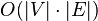$O(|V|\cdot |E|)$ , where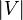$|V|$ and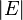$|E|$ are the number of vertices and edges
function BellmanFord(list vertices, list edges, vertex source)
::weight[],predecessor[]

// This implementation takes in a graph, represented as
// lists of vertices and edges, and fills two arrays
// (weight and predecessor) with shortest-path
// (less cost/weight/metric) information

// Step 1: initialize graph
for each vertex v in vertices:
if v is source then weight[v] := 0
else weight[v] := infinity
predecessor[v] := null

// Step 2: relax edges repeatedly
for i from 1 to size(vertices)-1:
for each edge (u, v) with weight w in edges:
if weight[u] + w < weight[v]:
weight[v] := weight[u] + w
predecessor[v] := u

// Step 3: check for negative-weight cycles
for each edge (u, v) with weight w in edges:
if weight[u] + w < weight[v]:
error "Graph contains a negative-weight cycle"
return weight[], predecessor[]

Java Code http://www.geekviewpoint.com/java/graph/bellman_ford_shortest_path
http://www.keithschwarz.com/interesting/code/bellman-ford/BellmanFord.java.html

Dijkstra’s shortest path algorithm - Priority QueueDijkstra's algorithm is an asymptotically the fastest known single-source shortest-path algorithm that solves the single-source shortest path problem for a graph with non-negative edge path costs, producing a shortest path tree.

Dijkstra’s algorithm is very similar to Prim’s algorithm for minimum spanning tree. Like Prim’s MST, we generate a SPT (shortest path tree) with given source as root. We maintain two sets, one set contains vertices included in shortest path tree, other set includes vertices not yet included in shortest path tree. At every step of the algorithm, we find a vertex which is in the other set (set of not yet included) and has minimum distance from source.

1) Create a set sptSet (shortest path tree set) that keeps track of vertices included in shortest path tree, i.e., whose minimum distance from source is calculated and finalized. Initially, this set is empty.
2) Assign a distance value to all vertices in the input graph. Initialize all distance values as INFINITE. Assign distance value as 0 for the source vertex so that it is picked first.
3) While sptSet doesn’t include all vertices
….a) Pick a vertex u which is not there in sptSetand has minimum distance value.
….b) Include u to sptSet.
….c) Update distance value of all adjacent vertices of u. To update the distance values, iterate through all adjacent vertices. For every adjacent vertex v, if sum of distance value of u (from source) and weight of edge u-v, is less than the distance value of v, then update the distance value of v.
Java Code
http://cs.fit.edu/~ryan/java/programs/graph/Dijkstra-java.html
http://www.algolist.com/code/java/Dijkstra%27s_algorithm
public static void computePaths(Vertex source) { source.minDistance = 0.; PriorityQueue vertexQueue = new PriorityQueue(); vertexQueue.add(source); while (!vertexQueue.isEmpty()) { Vertex u = vertexQueue.poll(); // Visit each edge exiting u for (Edge e : u.adjacencies) { Vertex v = e.target; double weight = e.weight; double distanceThroughU = u.minDistance + weight; if (distanceThroughU < v.minDistance) { vertexQueue.remove(v); v.minDistance = distanceThroughU ; v.previous = u; vertexQueue.add(v); } } } }

(DAG)Shortest Path in Directed Acyclic Graph
Time:O(V+E)
For a general weighted graph, we can calculate single source shortest distances in O(VE) time using Bellman–Ford Algorithm. For a graph with no negative weights, we can do better and calculate single source shortest distances in O(E + VLogV) time using Dijkstra’s algorithm.

Can we do even better for Directed Acyclic Graph (DAG)? We can calculate single source shortest distances in O(V+E) time for DAGs. The idea is to use Topological Sorting.
1) Initialize dist[] = {INF, INF, ….} and dist[s] = 0 where s is the source vertex.
2) Create a toplogical order of all vertices.
3) Do following for every vertex u in topological order.
………..Do following for every adjacent vertex v of u
………………if (dist[v] > dist[u] + weight(u, v))
………………………dist[v] = dist[u] + weight(u, v)

All-Pairs Shortest Paths(APSP)
1. All-Pairs shortest paths via fast matrix multiplication - O(N^3logN)

Dynamic Programming
Consider a graph G with vertices V numbered 1 through N. Further consider a function shortestPath(ijk) that returns the shortest possible path from i to j using vertices only from the set {1,2,...,k} as intermediate points along the way. Now, given this function, our goal is to find the shortest path from each i to each j using only vertices 1 to k + 1.

For each of these pairs of vertices, the true shortest path could be either (1) a path that only uses vertices in the set {1, ..., k} or (2) a path that goes from i to k + 1 and then from k + 1 to j. We know that the best path from i to j that only uses vertices 1 through k is defined by shortestPath(ijk), and it is clear that if there were a better path from i to k + 1 to j, then the length of this path would be the concatenation of the shortest path from i to k + 1 (using vertices in {1, ..., k}) and the shortest path from k + 1 to j (also using vertices in {1, ..., k}).

If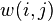$w(i, j)$ is the weight of the edge between vertices i and j, we can define shortestPath(ijk + 1) in terms of the following recursive formula: the base case is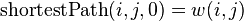$\textrm{shortestPath}(i, j, 0) = w(i, j)$
and the recursive case is$\textrm{shortestPath}(i,j,k+1) = \min(\textrm{shortestPath}(i,j,k),\,\textrm{shortestPath}(i,k+1,k) + \textrm{shortestPath}(k+1,j,k))$
Path reconstruction
let dist be a |V| × |V| array of minimum distances initialized to ∞ (infinity)
let next be a |V| × |V| array of vertex indices initialized to null

procedure FloydWarshallWithPathReconstruction ()
for each edge (u,v)
dist[u][v] ← w(u,v)  // the weight of the edge (u,v)
next[u][v] ← v
for k from 1 to |V| // standard Floyd-Warshall implementation
for i from 1 to |V|
for j from 1 to |V|
if dist[i][k] + dist[k][j] < dist[i][j] then
dist[i][j] ← dist[i][k] + dist[k][j]
next[i][j] ← next[i][k]

procedure Path(u, v)
if next[u][v] = null then
return []
path = [u]
while u ≠ v
u ← next[u][v]
path.append(u)
return path
Dynamic Programming | Set 16 (Floyd Warshall Algorithm) - GeeksforGeeksJava
http://cs.anu.edu.au/people/Alistair.Rendell/Teaching/apac_comp3600/module4/all_pairs_shortest_paths.xhtml
code: http://algs4.cs.princeton.edu/44sp/FloydWarshall.java.html

Maximum Flow
Ford Fulkerson Method
Algorithm Ford–Fulkerson
Inputs Given a Network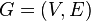$G = (V,E)$ with flow capacity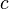$c$, a source node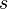$s$, and a sink node$t$
Output Compute a flow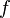$f$ from$s$ to$t$ of maximum value
1.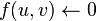$f(u,v) \leftarrow 0$ for all edges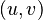$(u,v)$
2. While there is a path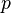$p$ from$s$ to$t$ in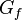$G_f$, such that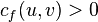$c_f(u,v) > 0$ for all edges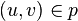$(u,v) \in p$:
1. Find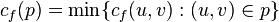$c_f(p) = \min\{c_f(u,v) : (u,v) \in p\}$
2. For each edge$(u,v) \in p$
1.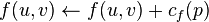$f(u,v) \leftarrow f(u,v) + c_f(p)$ (Send flow along the path)
2.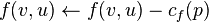$f(v,u) \leftarrow f(v,u) - c_f(p)$ (The flow might be "returned" later)
http://blog.csdn.net/smartxxyx/article/details/9293665
Code: http://chuanwang66.iteye.com/blog/1446977

Edmonds–Karp algorithm
It's an implementation of the Ford–Fulkerson method for computing the maximum flow in a flow network in O(V E2) time.

The algorithm is identical to the Ford–Fulkerson algorithm except that it uses BFS to find the augmenting path

Java code: http://en.wikibooks.org/wiki/Algorithm_Implementation/Graphs/Maximum_flow/Edmonds-Karp
/**
* Finds the maximum flow in a flow network.
* @param E neighbour lists
* @param C capacity matrix (must be n by n)
* @param s source
* @param t sink
* @return maximum flow
*/
public class EdmondsKarp {
public static int edmondsKarp(int[][] E, int[][] C, int s, int t) {
int n = C.length;
// Residual capacity from u to v is C[u][v] - F[u][v]
int[][] F = new int[n][n];
while (true) {
int[] P = new int[n]; // Parent table
Arrays.fill(P, -1);
P[s] = s;
int[] M = new int[n]; // Capacity of path to node
M[s] = Integer.MAX_VALUE;
// BFS queue
Queue<Integer> Q = new LinkedList<Integer>();
Q.offer(s);
LOOP:
while (!Q.isEmpty()) {
int u = Q.poll();
for (int v : E[u]) {
// There is available capacity,
// and v is not seen before in search
if (C[u][v] - F[u][v] > 0 && P[v] == -1) {
P[v] = u;
M[v] = Math.min(M[u], C[u][v] - F[u][v]);
if (v != t)
Q.offer(v);
else {
// Backtrack search, and write flow
while (P[v] != v) {
u = P[v];
F[u][v] += M[t];
F[v][u] -= M[t];
v = u;
}
break LOOP;
}
}
}
}
if (P[t] == -1) { // We did not find a path to t
int sum = 0;
for (int x : F[s])
sum += x;
return sum;
}
}
}
}

## Labels

adsense (5) Algorithm (69) Algorithm Series (35) Android (4) ANT (6) bat (8) Big Data (7) Blogger (14) Bugs (4) Cache (5) Chrome (17) Code Example (29) Code Quality (6) Concurrency (4) Database (7) Debug (16) Design (5) Dev Tips (62) Eclipse (32) GAE (4) Git (5) Google (27) Guava (7) How to (9) Http Client (8) IDE (6) Interview (88) J2EE (13) J2SE (49) Jackson (4) Java (177) JavaScript (27) JSON (7) Linux (22) Lucene-Solr (112) Mac (10) Maven (8) Memory Usage (4) Network (9) Nutch2 (18) OpenNLP (4) Performance (9) PowerShell (11) Problem Solving (11) regex (5) Review (4) Scala (6) Security (9) Soft Skills (38) Spark (4) Spring (22) System Design (11) Testing (6) Text Mining (14) Tips (12) Tools (24) Troubleshooting (29) UIMA (9) Web Development (19) Windows (21) xml (5)